# ML Aggarwal Class 10 Solutions for ICSE Maths Chapter 15 Circles Chapter Test

## ML Aggarwal Class 10 Solutions for ICSE Maths Chapter 15 Circles Chapter Test

ML Aggarwal Class 10 Solutions for ICSE Maths Chapter 15 Circles Chapter Test

Question 1.
(a) In the figure (i) given below, triangle ABC is equilateral. Find ∠BDC and ∠BEC.
(b) In the figure (ii) given below, AB is a diameter of a circle with centre O. OD is perpendicular to AB and C is a point on the arc DB. Find ∠BAD and ∠ACDSolution: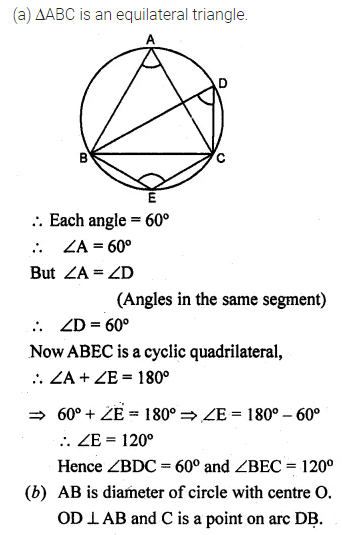Question 2.
(a) In the figure given below, AB is a diameter of the circle. If AE = BE and ∠ADC = 118°, find
(i) ∠BDC (ii) ∠CAE.(b) In the figure given below, AB is the diameter of the semi-circle ABCDE with centre O. If AE = ED and ∠BCD = 140°, find ∠AED and ∠EBD. Also, Prove that OE is parallel to BD.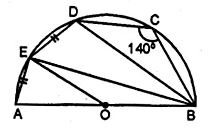Solution: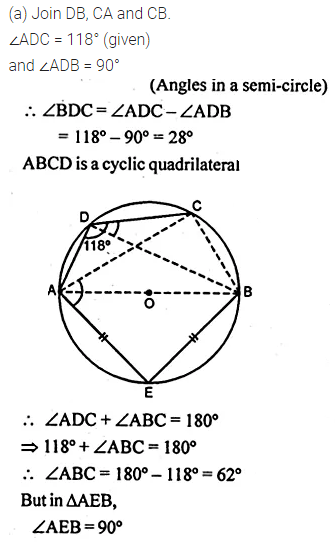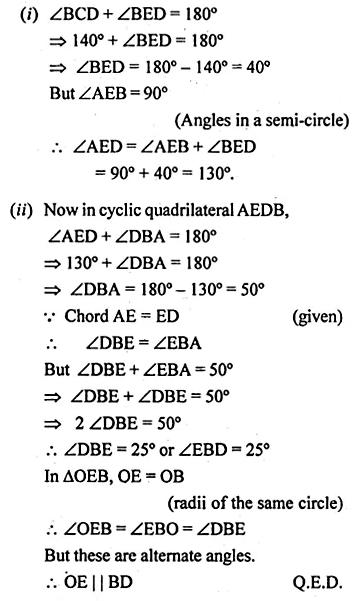Question 3.
(a) In the figure (i) given below, O is the centre of the circle. Prove that ∠AOC = 2 (∠ACB + ∠BAC).
(b) In the figure (ii) given below, O is the centre of the circle. Prove that x + y = z.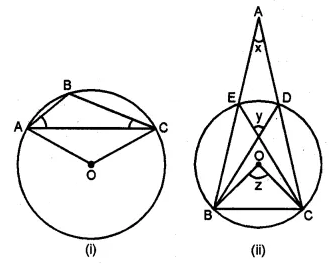Solution: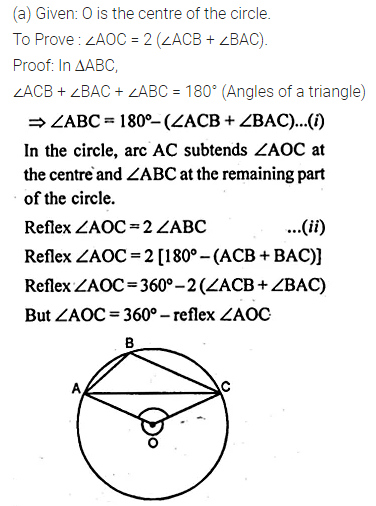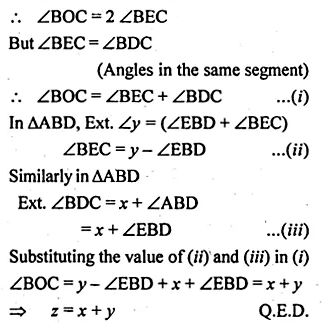Question 4.
(a) In the figure (i) given below, AB is the diameter of a circle. If DC is parallel to AB and ∠CAB = 25°, find:
(i)∠ADC
(ii) ∠DAC.
(b) In the figure (ii) given below, the centre O of the smaller circle lies on the circumference of the bigger circle. If ∠APB = 70° and ∠BCD = 60°, find:
(i) ∠AOB
(ii) ∠ACB
(iii) ∠ABD
(iv) ∠ADB.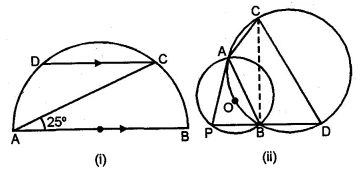Solution: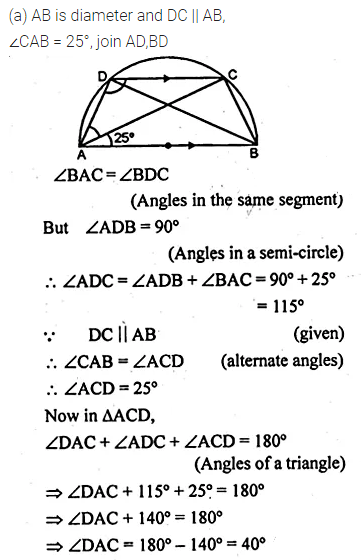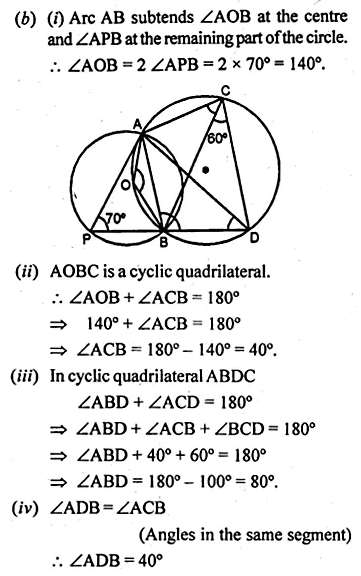Question 5.
(a) In the figure (i) given below, ABCD is a cyclic quadrilateral. If AB = CD, Prove that AD = BC.
(b) In the figure (ii) given below, ABC is an isosceles triangle with AB = AC. If ∠ABC = 50°, find ∠BDC and ∠BEC.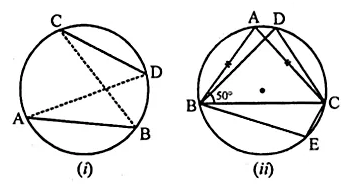Solution: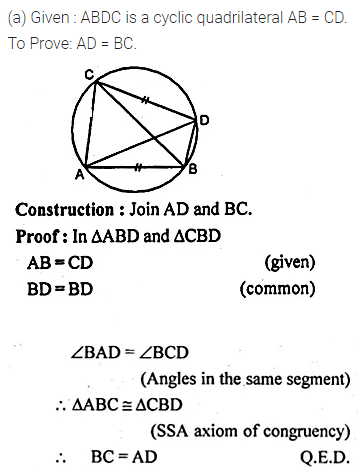Question 6.
A point P is 13 cm from the centre of a circle. The length of the tangent drawn from P to the circle is 12 cm. Find the distance of P from the nearest point of the circle.
Solution: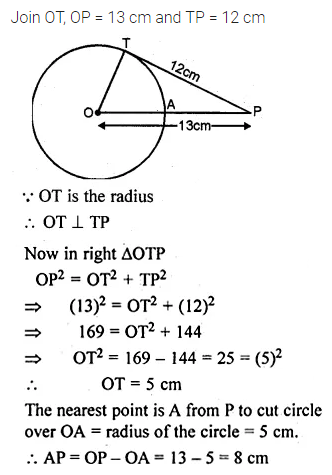Question 7.
Two circles touch each other internally. Prove that the tangents drawn to the two circles from any point on the common tangent are equal in length.
Solution: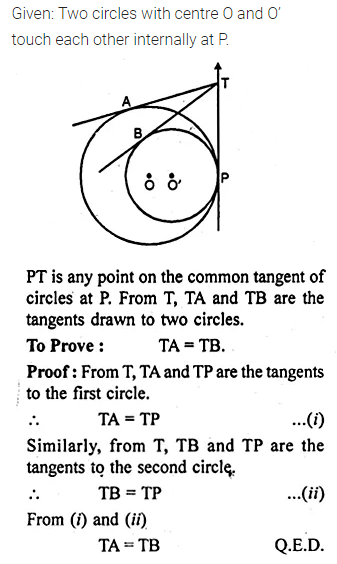Question 8.
From a point outside a circle, with centre O, tangents PA and PB are drawn. Prove that
(i) ∠AOP = ∠BOP.
(ii) OP is the perpendicular bisector of the chord AB.
Solution: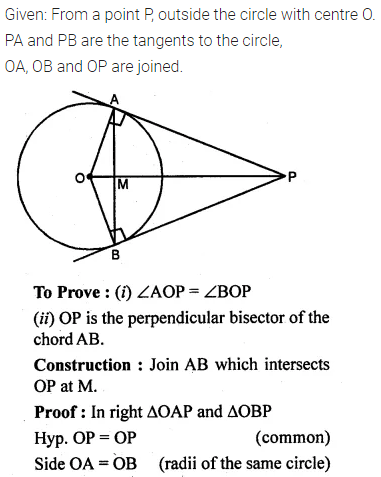Question 9.
(a) The figure given below shows two circles with centres A, B and a transverse common tangent to these circles meet the straight line AB in C. Prove that:
AP : BQ = PC : CQ.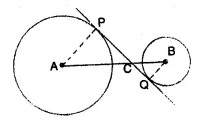(b) In the figure (ii) given below, PQ is a tangent to the circle with centre O and AB is a diameter of the circle. If QA is parallel to PO, prove that PB is tangent to the circle.Solution: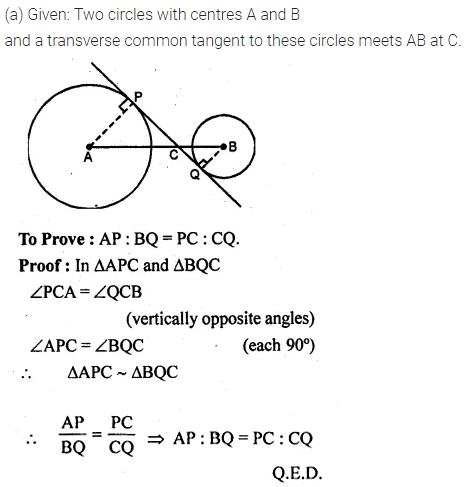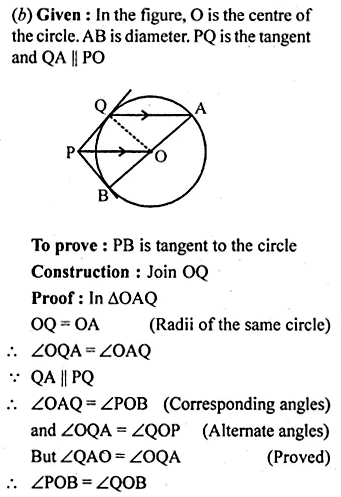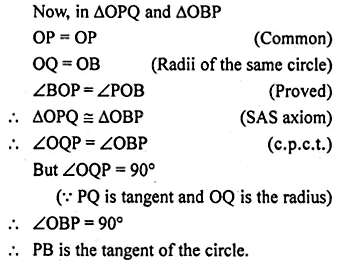Question 10.
In the figure given below, two circles with centres A and B touch externally. PM is a tangent to the circle with centre A and QN is a tangent to the circle with centre B. If PM = 15 cm, QN = 12 cm, PA = 17 cm and QB = 13 cm, then find the distance between the centres A and B of the circles.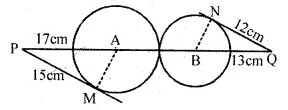Solution:Question 11.
Two chords AB, CD of a circle intersect externally at a point P. If PB = 7 cm, AB = 9 cm and PD = 6 cm, find CD.
Solution: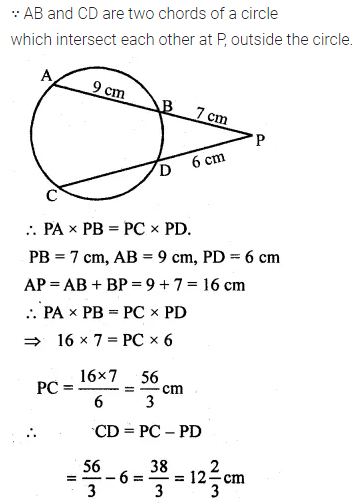Question 12.
(a) In the figure (i) given below, chord AB and diameter CD of a circle with centre O meet at P. PT is tangent to the circle at T. If AP = 16 cm, AB = 12 cm and DP = 2 cm, find the length of PT and the radius of the circle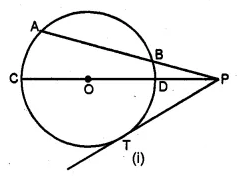(b) In the figure (ii) given below, chord AB and diameter CD of a circle meet at P. If AB = 8 cm, BP = 6 cm and PD = 4 cm, find the radius of the circle. Also, find the length of the tangent drawn from P to the circle.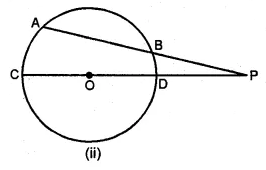Solution:Question 13.
In the figure given below, chord AB and diameter PQ of a circle with centre O meet at X. If BX = 5 cm, OX = 10 cm and.the radius of the circle is 6 cm, compute the length of AB. Also, find the length of tangent drawn from X to the circle.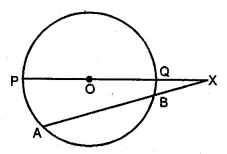Solution: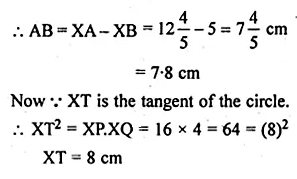Question 14.
(a) In the figure (i) given below, ∠CBP = 40°, ∠CPB = q° and ∠DAB = p°. Obtain an equation connecting p and q. If AC and BD meet at Q so that ∠AQD = 2 q° and the points C, P, B and Q are concyclic, find the values of p and q.
(b) In the figure (ii) given below, AC is a diameter of the circle with centre O. If CD || BE, ∠AOB = 130° and ∠ACE = 20°, find:
(i)∠BEC
(ii) ∠ACB
(iii) ∠BCD
(iv) ∠CED.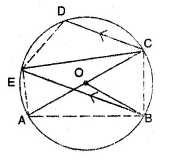Solution: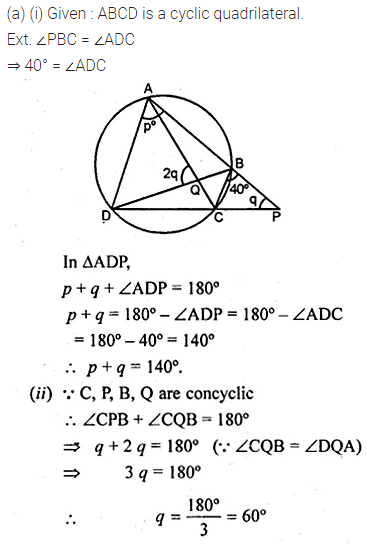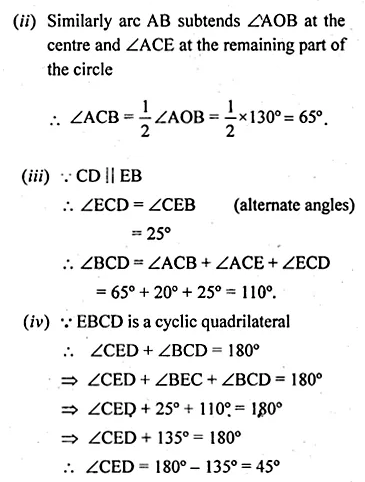Question 15.
(a) In the figure (i) given below, APC, AQB and BPD are straight lines.
(i) Prove that ∠ADB + ∠ACB = 180°.
(ii) If a circle can be drawn through A, B, C and D, Prove that it has AB as a diameter(b) In the figure (ii) given below, AQB is a straight line. Sides AC and BC of ∆ABC cut the circles at E and D respectively. Prove that the points C, E, P and D are concyclic.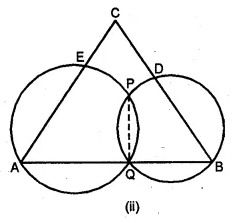Solution: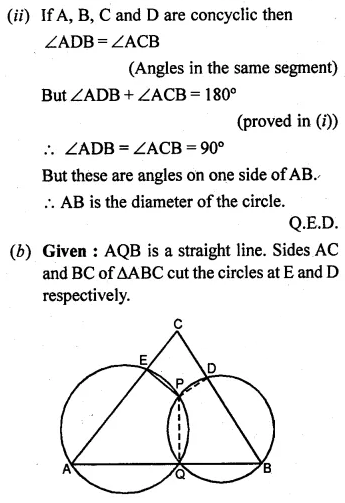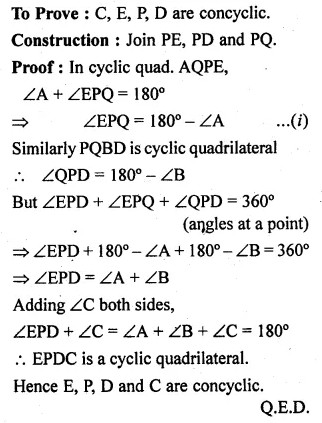Question 16.
(a) In the figure (i) given below, chords AB, BC and CD of a circle with centre O are equal. If ∠BCD = 120°, find
(i) ∠BDC
(ii) ∠BEC
(iii) ∠AEC
(iv) ∠AOB.
Hence Prove that AOAB is equilateral.
(b) In the figure (ii) given below, AB is a diameter of a circle with centre O. The chord BC of the circle is parallel to the radius OD and the lines OC and BD meet at E. Prove that
(i) ∠CED = 3 ∠CBD
(ii) CD = DA.Solution: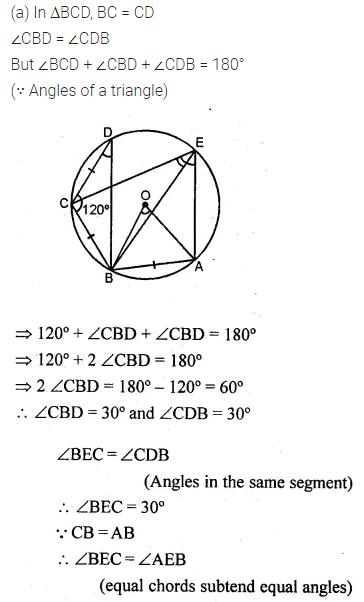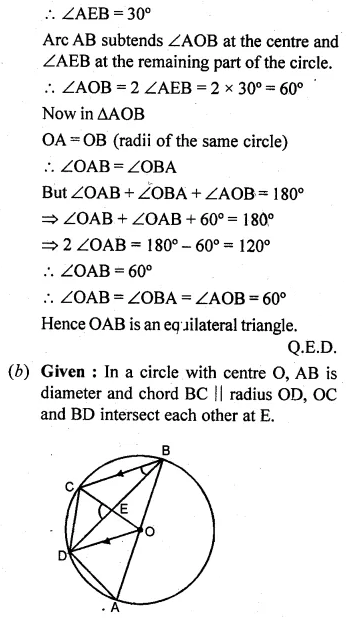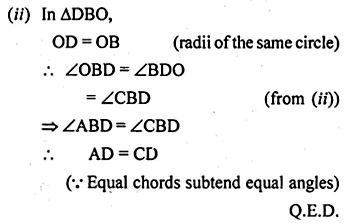Question 17.
(a) In the adjoining figure, (i) given below AB and XY are diameters of a circle with centre O. If ∠APX = 30°, find
(i) ∠AOX
(ii) ∠APY
(iii) ∠BPY
(iv) ∠OAX.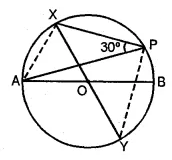(b) In the figure (ii) given below, AP and BP are tangents to the circle with centre O. If ∠CBP = 25° and ∠CAP = 40°, find :
(i) ∠ADB
(ii) ∠AOB
(iii) ∠ACB
(iv) ∠APB.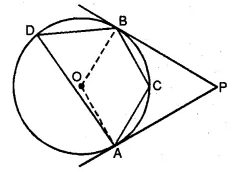Solution: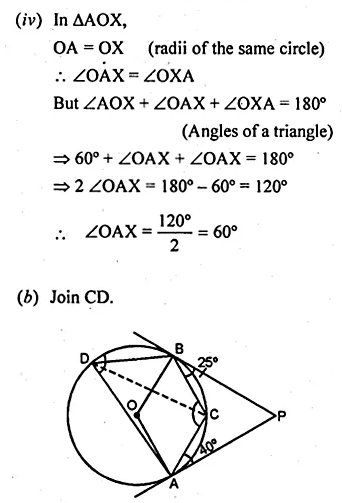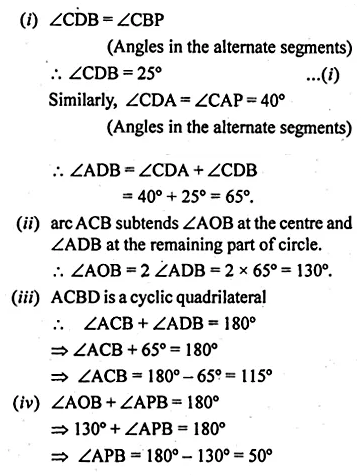ML Aggarwal Class 10 Solutions for ICSE Maths